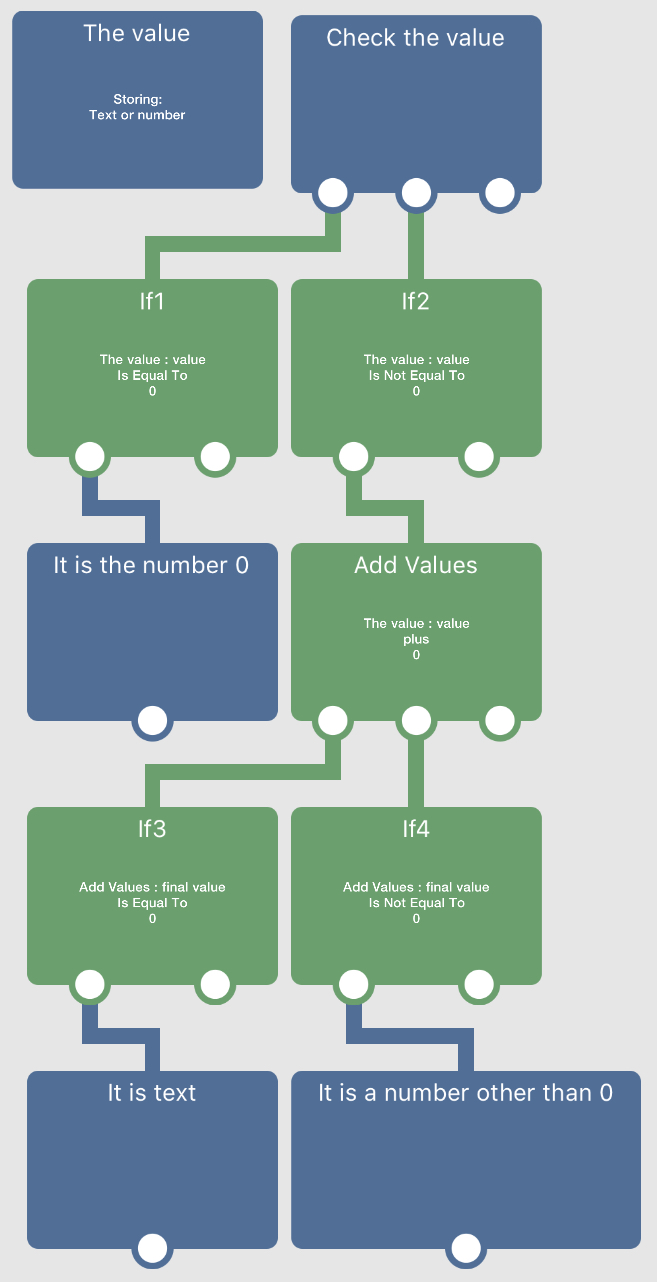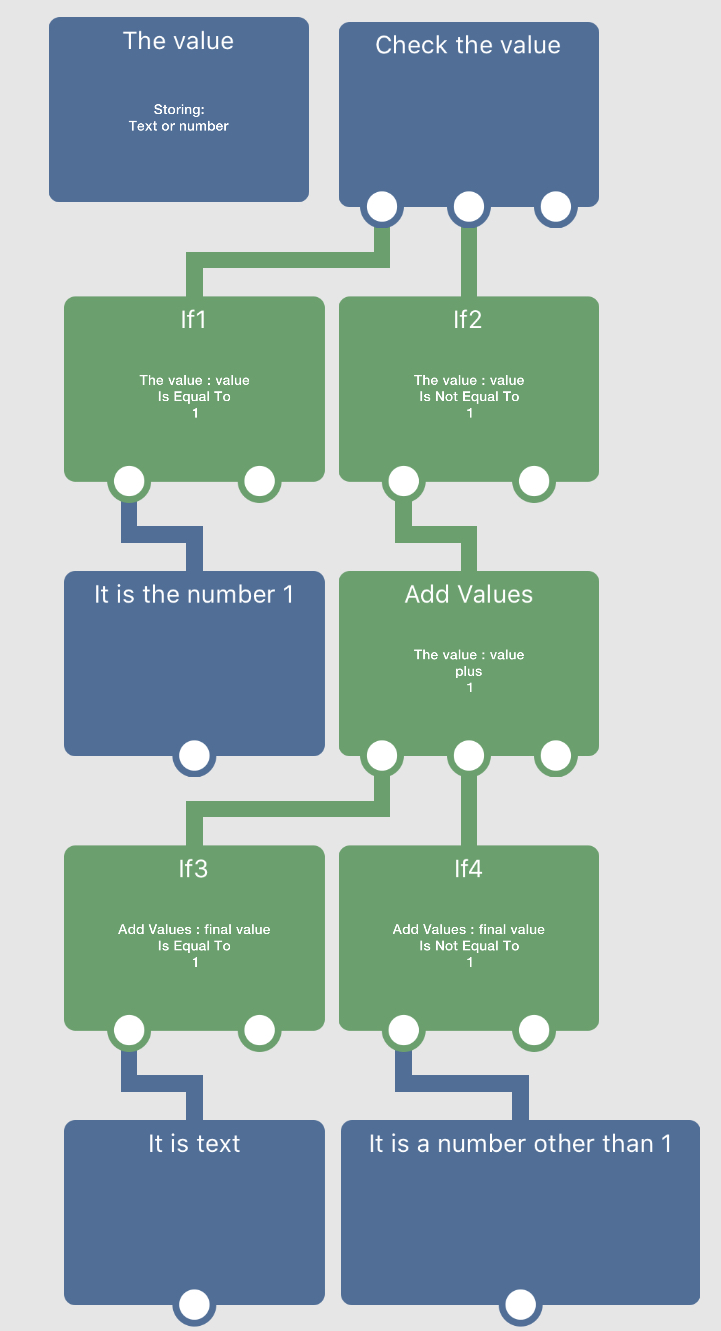# What's the best way to check if input is not a number

• Anyone know of a good way to check if some input is a number or not?

• @kamdroid add 1 to the input and if the add outputs a 1 it’s text. If it isn’t 1 it is a number.

• @itap-development Or it's zero 😜

• @kamdroid Picture of basically what @iTap-Development said, put into behaviours (except I'm using 0 rather than 1)• Just realized this won’t work if the input is already the number 0 though lol. You would still get the output of 1 (or 0).
But another if would fix!

• @aidan-oxley NOOOOOOO!!! You have to use ONE! 🤨🤨🤨 🤪🤪🤪

• This post is deleted!

• @iTap-Development @Aidan-Oxley Thank you, this is a nice solution.

edit: I just released my simple calculator, which is why I wanted to ask this question. https://www.hyperpad.com/projects/asudb1ye

• @kamdroid Cool, I will check it out because it sounds interesting!

• @itap-development 🙄EDIT: this does not work, use the other one somewhere above.

• @aidan-oxley those first 2 ifs should be checking if it's zero, right? Because text+1 is the same as 0+1?

• @jack8680 First it checks whether the value is the number 1 or not, if it is then it's done and it's a number. If it's not the number 1, then it adds 1 to it. If that result is 1, then it was text (since text will be treated as zero, so 0 + 1 = 1), if the result was not 1, then it is any number other than 1 (since 1 was already checked for at the start). I prefer adding 0 rather than 1 lol.

• Just realized this won’t work if the input is already the number 0 though lol. You would still get the output of 1 (or 0).
But another if would fix!

🤦‍♂️ just realized your first screen shot already did this... I’m stupid🤯

• I thought the first if statements should check if it is 0, not 1, too.

If input was 0, 0+1 = 1, creating a false-positive to it being not a valid number.

If input was 1, and the if statements didn't exist, 1+1 = 2, this would say it is a valid number.

• Ah ok, yeah the second one I made just to satisfy @iTap-Development's OCD wouldnt work, the first set of Ifs probably needed to stay as zero. Just use the one that adds zero lol.

• Whether you add 1 or 0 you still need all the ifs in both screen shots, right?